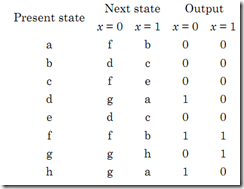### DIGITAL LOGIC CIRCUITS–April / May 2010 Question Paper

Anna University

B.E./B.Tech. DEGREE EXAMINATION, APRIL/MAY 2010

Fourth Semester

Electrical and Electronics Engineering

EE2255 — DIGITAL LOGIC CIRCUITS

(Regulation 2008)

Time: Three hours

Maximum: 100 Marks

PART A — (10 × 2 = 20 Marks)

1. Show that

(a) a + a'b = a + b

(b) x' y' z + x' yz + xy' = x' z + xy' .

2. Draw the Truth table and logic circuit of half adder.

3. Draw the circuit of SR Flip flop.

4. What are synchronous sequential circuits?

5. Give the characteristic equation and state diagram of JK flip flop.

6. What is a self starting counter?

7. What is the advantage of PLA over ROM?

8. Which IC family offers (a) low propagation delay, and (b)low power dissipation?

9. Write HDL for half adder.

10. What are the various modeling techniques in HDL?

PART B — (5 × 16 = 80 Marks)

11. (a) (i) Simplify using k-map

F (w, x, y, z ) = ∑ (0,1,2,4,5,6,8,9,12,13,14) . (8)

(ii) Design a BCD to Excess-3 code converter. (8)

Or

(b) (i) Solve g (w, x, y, z ) = ∑ m (1,3,4,6,11) + ∑ d (0,8,10,12,13) . (8)

12. (a) Design a synchronous sequential circuit using JK for the given state diagram. (16)Or

(b) Design a BCD counter using T flip flop. (16)

13. (a) Design BCD ripple counter using JK flip flop. (16) Or

(b) (i) Reduce the number of states in the following state table. (12) Next state Output(ii) Starting from a, find the output sequence generated with input sequence 01110010011. (4)

 14. (a) (i) Design a combinatorial circuit using ROM. The circuit accepts 3-bit number and generates an output binary number equal to square of input number. (8) (ii) Repeat the above problem using PLA. (8)

Or

(b) (i) Compare all the IC logic families based on

(1) Power consumption

(2) Fan out

(3) Power dissipation (4) Propagation delay (5) Switching speed

(6) Noise margin. (8)

(ii) Describe the different types of memories. (8)

15. (a) Write HDL program for full adder and 4 bit comparator. (16)

Or

 (b) (i) Write an HDL behavioral description of JK flip flop using statement based on the value of present state. if-else (8) (ii) Draw the logic diagram for the following module. (8) module seqcrt (A, B, C, Q, CLK) ; input A, B, C, CLK ; output Q ; reg Q, E ; always @ (Posedge CLK) begin E < = A & B ; Q < = E/C ; end end module

————––––—